## Filters

Sort by :
Clear All
Q

Two lenses of power –15 D and +5 D are in
contact with each other. The focal length of the
combination is

• Option 1)

+10cm

• Option 2)

-20cm

• Option 3)

-10cm

• Option 4)

+20cm

Option 1) +10cm Option 2) -20cm Option 3) -10cm Option 4) +20cm

The refractive index of glass is 1.520 for red light and 1.525 for blue light. Let D1 and D2 be an of minimum deviation for red and blue light respectively in a prism of this glass. Then

• Option 1)

D1>D2

• Option 2)

D1<D2

• Option 3)

D1=D2

• Option 4)

D1 can be less than or greater than depending upon the angle of prism

Option 1) D1>D2 Option 2) D1<D2 Option 3) D1=D2 Option 4) D1 can be less than or greater than depending upon the angle of prism

A monochromatic light is incident at a certain angle on an equilateral triangular prism and suffers minimum deviation. If the refractive index of the material of the prism is , then the angle of incidence is:

• Option 1)

• Option 2)

• Option 3)

• Option 4)

Relation between angle of incidence and angle of refaction -   - wherein refractive index of medium of incidence.   refractive index of medium where rays is refracted. angle of incidence. angle of refraction. For minimum deviation  &  apply snell law  Option 1)   Option 2)  Option 3)  Option 4)

In a double-slit experiment, green light (5303 ) falls on a double slit having a separation of 19.44 and a width of 4.05 . The number of bright fringes between the first and the second diffraction minima is :

• Option 1)

04

• Option 2)

10

• Option 3)

09

• Option 4)

05

Young Double Slit Experiment - - wherein Distance of a point on screen from central maxima Path difference at that point                                                                            In YDS  For diffraction  For 1st minima For 2nd minima Now for Interference . Path difference at  Path difference at  So order of maxima between A & B  So 5 bright fringes will present between...

An object is at distance of 20m from a convex lens of focal length 0.3m. The lens forms an image of the object moves away from the lens at a speed of 5m/s, the speed and direction of the image will be:

• Option 1)

3.22 X 10-3 m/s towards the lens

• Option 2)

towards the lens

• Option 3)

away from the lens

• Option 4)

away from the lens

Relation between object and image velocity if object is moving along principal axis - = - = -           - wherein = velocity of object = velocity of image = velocity of mirror = velocity of object w.r.t. mirror = velocity of image w.r.t. mirror From lens formula  Velocity of image w.r.to lens and directions is same as that of object towards the lens.    Option 1)3.22 X...

The variation of refractive index of crown glass thin prism with wavelength of the incident light is shown. Which of the following graphs is the correct one, if Dm is the angle of minimum deviation?• Option 1)• Option 2)• Option 3)• Option 4)Angle of deviation through prism (δ) - - wherein angle of incidence angle of emergence angle of prism      Option 1)Option 2)Option 3)  Option 4)

In a Young's double slit experiment, the path difference , at a certain point on the screen between two interfering waves is of the wavelength. The ratio of the intensity at this point to that at the centre of a bright fringe is close to:

• Option 1)

0.80

• Option 2)

0.94

• Option 3)

0.85

• Option 4)

0.74

Resultant Intensity of two wave - - wherein Intencity of wave 1 Intencity of wave 2 Phase difference      Option 1)0.80Option 2)0.94Option 3)0.85Option 4)0.74

Formation of real image using a biconvex lens is shown below :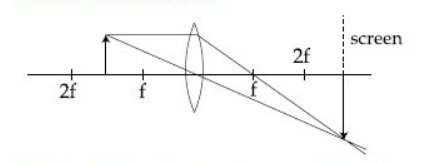If the whole set up is immersed in water without disturbing the object and the screen positions , what will one observe on the screen ?

• Option 1)

Erect real image

• Option 2)

Image disappears

• Option 3)

Magnified image

• Option 4)

No change

Lensmaker's Formula -   - wherein refractive index of medium of object refractive index of lens are radius of curvature of two surface   We have and If we change urel then f will change. If f will change for then for same u value. V value will change but screen position is not changed so image will disaapear.  Option 1)Erect real imageOption 2)Image disappearsOption 3)Magnified...

A plano-convex lens (focal length f2 , refractive index , radius of curvature R ) fits exactly into a plano-concave lens ( focal length f1 , refractive index , radius of curvature R ) . Their plane surfaces are parallel to each other. Then , the focal length of the combination will be :

• Option 1)

• Option 2)

f1 + f2

• Option 3)

f1 - f2

• Option 4)

Lensmaker's Formula -   - wherein refractive index of medium of object refractive index of lens are radius of curvature of two surface and  Option 1)Option 2) f1 + f2Option 3) f1 - f2Option 4)

The eye can be regarded as a single refracting surface. The radius of curvature of this surface is equal to that of cornea (7.8mm) . This surface separates two media of refractive indices 1 and 1.34. Calculate the distance from the refracting surface at which a parallel beam of light will come to focus.

• Option 1)

• Option 2)

• Option 3)

• Option 4)

Refraction through spherical surface -   - wherein Object distance image distance Radius of curvature   Option 1)  Option 2)Option 3)Option 4)

Consider a Young's double slit experiment as shown in figure. What should be the slit sepration d in terms of wavelength such that the first minima occurs directly in front of the slit (S1)?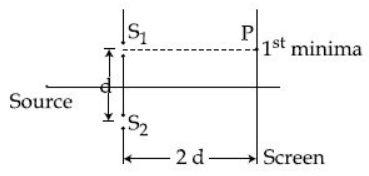• Option 1)

• Option 2)

• Option 3)

• Option 4)

Minimum amplitude & Intensity -   - wherein     Young Double Slit Experiment - - wherein Distance of a point on screen from central maxima Path difference at that point   x1 = 2d,    Option 1)Option 2)Option 3)Option 4)

A plano lens of refractive index  and focal length  is kept in contact with another plano concave lens of refractive index  and focal length . If the radius of curvature of their spherical faces is R each and  then  and  are related as:

• Option 1)• Option 2)• Option 3)• Option 4)Lensmaker's Formula -   - wherein refractive index of medium of object refractive index of lens are radius of curvature of two surface for lens 1 we have for lens 2   and from (1),(2) and (3) we get    Option 1)Option 2)Option 3)Option 4)

In a young's double slit experiment with slit sepration 0.1mm, one observes a bright fringe at angle rad by using light of wavelength . When the light of wavelength is used a bright fringe is seen at the same angle in the same set up. Given that and are in visible range (380nm to 740nm), their values are:

• Option 1)

625 nm, 500nm

• Option 2)

380 nm, 525 nm

• Option 3)

380 nm, 500 nm

• Option 4)

400 nm, 500 nm

Young Double Slit Experiment - - wherein Distance of a point on screen from central maxima Path difference at that point        Option 1)625 nm, 500nmOption 2)380 nm, 525 nmOption 3)380 nm, 500 nmOption 4)400 nm, 500 nm

Two plane mirrors are inclined to each other such that a ray of light incident on the first mirror (M1) and parallel to the second mirror (M2) is finally reflect from the second mirror (M2) parallel to the first mirror (M1). The angle between the two mirrors will be:

• Option 1)

• Option 2)

• Option 3)

• Option 4)

Law of reflection - 1)    Incident ray, reflected rays and normal at the point of incidence lie in the same plane. 2)    Angle of incidence is equal to angle of reflection. - wherein                    Option 1)Option 2)Option 3)Option 4)

In a Young's double slit experiment, the slits are placed 0.320 mm apart. Light of wavelength = 500nm is incident on the slits. The total number of bright fringes that are observed in the angular range is:

• Option 1)

320

• Option 2)

321

• Option 3)

640

• Option 4)

641

Young Double Slit Experiment - - wherein Distance of a point on screen from central maxima Path difference at that point Path difference   separation of slits  number of maximas from                                                                      Option 1)320Option 2)321Option 3)640Option 4)641

A point source of light, S is placed at a distance L in front of the centre of plane mirror of width d which is hanging vertically on a wall. A man walks in front of the mirror along a line parallel to the mirror., at a distance 2L as shown below . The distance over which the man can see the image of the light source in the mirror is :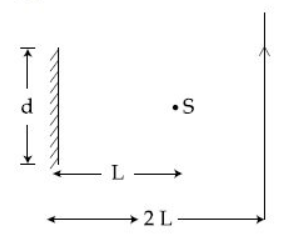• Option 1)

3d

• Option 2)

d

• Option 3)

• Option 4)

2d

Image formation from plane mirror - 1)    Distance of object from mirror = Distance of image from the mirror. 2)    Line joining a point object and its image is normal to the reflecting surface 3)    Size of image is same as that of the object. 4)    For real object the image is virtual and for a virtual object the image is real. - This question can be easily solved with help of...

What is the position and nature of image formed by lens combination shown in figure ? (f1, f2 are focal lengths )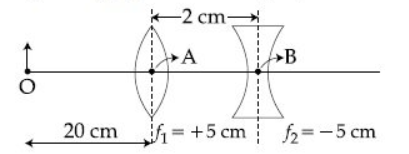• Option 1)

40 cm from point B at right; real

• Option 2)

from point B at right; real

• Option 3)

70 cm from point B at right; real

• Option 4)

70 cm from point B at left; virtual

Thin lens formula -   - wherein are object and image distance from lens. For first lens so,  So    for 2nd  lens is given by So   So      and image is real  Option 1)40 cm from point B at right; real Option 2)        from point B at right; real Option 3) 70 cm from point B at right; real Option 4)70 cm from point B at left; virtual

Consider a tank made of glass (refractive index 1.5) with a thick bottom. It is filled with a liquid of refractive index . A student finds that, irrespective of what the incident angle (see figure) is for a beam of light entering the liquid,the light reflected from the liquidglass interface is never completely polarized. For this to happen,the minimum value of is :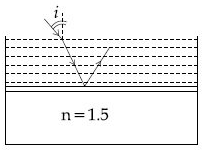• Option 1)

• Option 2)

• Option 3)

• Option 4)

Critical angle - = Refractive index of rarer medium / Refractive index of denser medium =  - wherein When angle of incidence of a travelling from a dence medium to rarer medium is greater than critical angle, no refraction occurs.   C < ib ib brewster angle C critical angle sin C < sin ib  For air liquid sin 90 = sin C since tan ib = sin C < sin ib Option 1)Option 2)Option 3)Option 4)

Two coherent sources produce waves of different intensities which interfere. After interference, the ratio of the maximum intensity to the minimum intensity is 16. The intensity of the waves are in the ratio:

• Option 1)

16 : 9

• Option 2)

25 : 9

• Option 3)

4 : 1

• Option 4)

5 : 3

Resultant Intensity of two wave - - wherein Intencity of wave 1 Intencity of wave 2 Phase difference Let intensities be I1, I2 and amplitudes A1,A2 Amax = A1 + A2 Amin = A1 - A2    Option 1)16 : 9Option 2)25 : 9  Option 3)4 : 1  Option 4)5 : 3

A convex lens is put 10cm from a light source and it makes sharp images on a screen, kept 10 cm from the lens. Now a glass block (refractive index 1.5) of 1.5 cm thickness is placed in contact with the light source. To get the sharp image again, the screen is shifted by a distance d. Then d is:

• Option 1)

11 cm away from the lens

• Option 2)

0

• Option 3)

0.55 cm towards the lens

• Option 4)

0.55 cm away from the lens

Refraction through parallel slab -   - wherein  shifting of object from slab thickness of slab Refractive Index of slab. F = = 5 cm Due to glass plate shift s = t = 1.5 = 0.5 New u =9.5 cm Using lens formula = v = 10.55 cm Shift  is 0.55 cm away from the lens.  Option 1)11 cm away from the lensOption 2)0Option 3)0.55 cm towards the lensOption 4)0.55 cm away from the lens
Exams
Articles
Questions